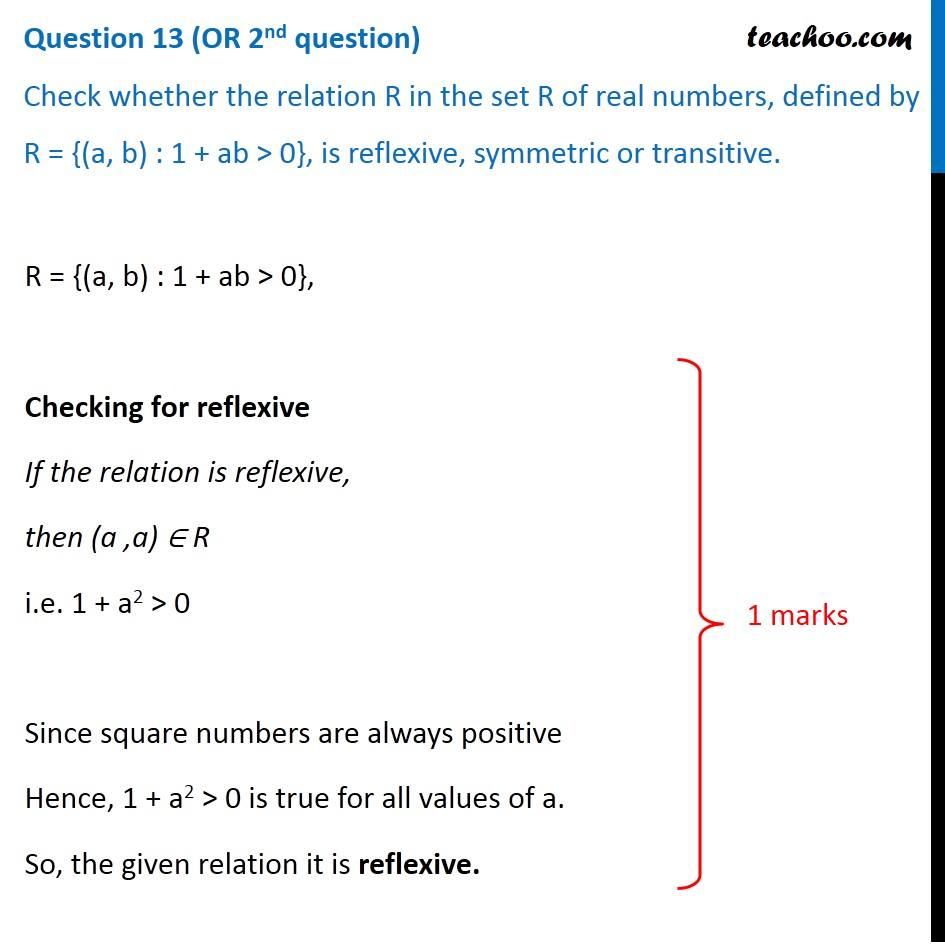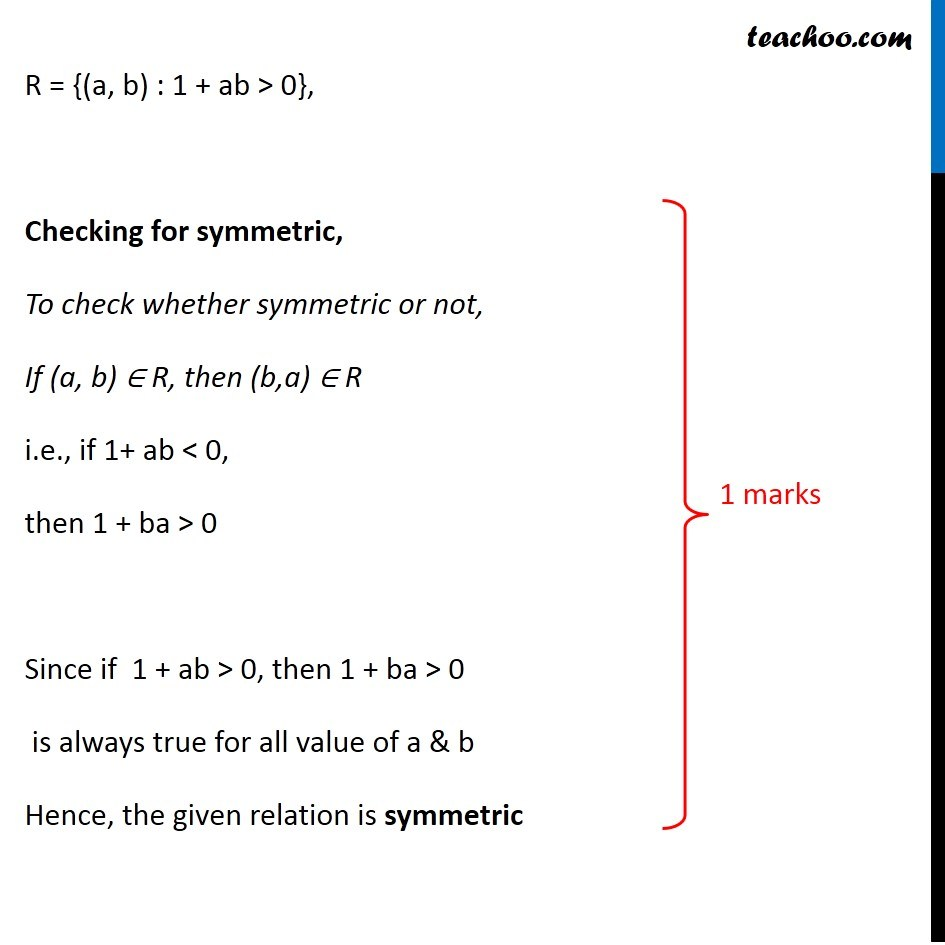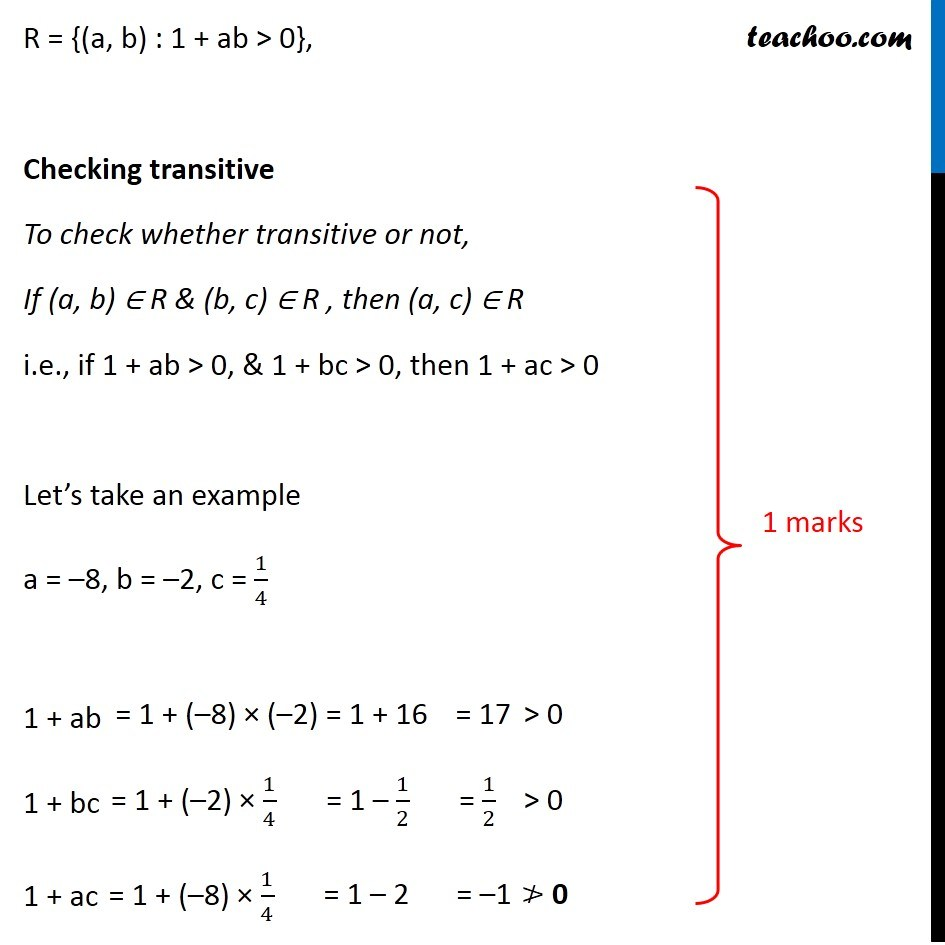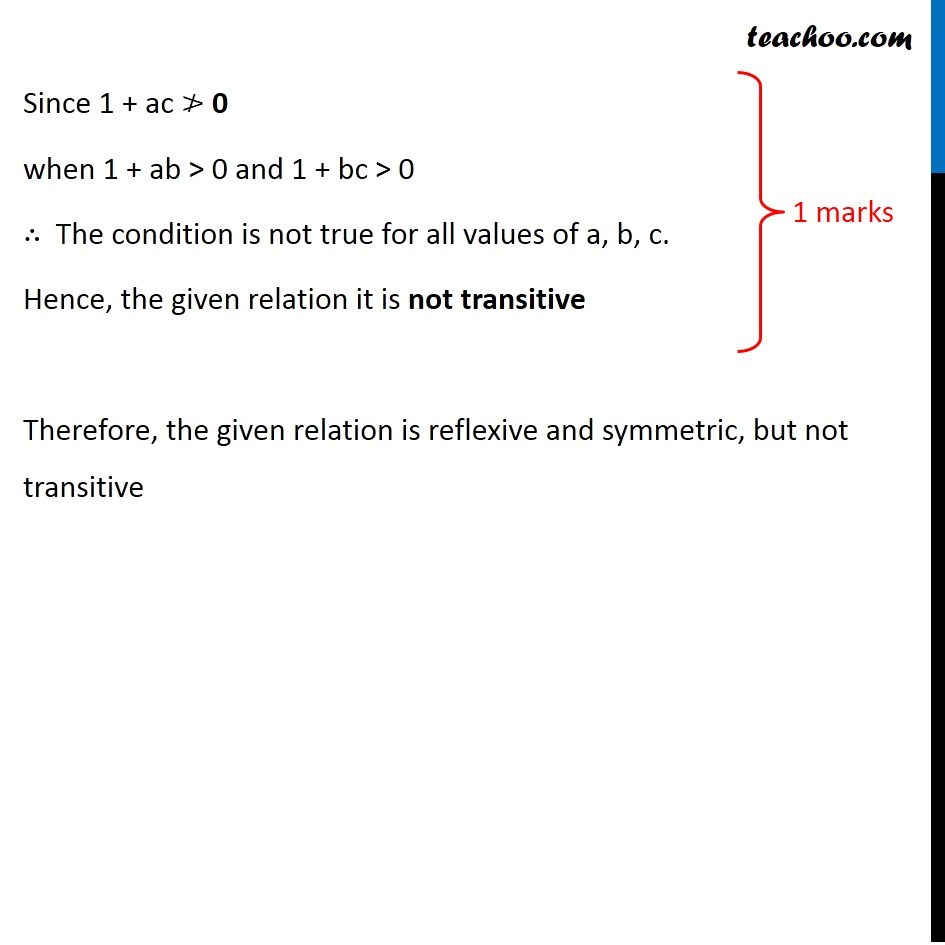CBSE Class 12 Sample Paper for 2019 Boards

Class 12
Solutions of Sample Papers and Past Year Papers - for Class 12 Boards

Question 13 (OR 2 nd question)

Check whether the relation R in the set R of real numbers, defined by R = {(a, b) : 1 + ab > 0}, is reflexive, symmetric or transitive.Learn in your speed, with individual attention - Teachoo Maths 1-on-1 Class

### Transcript

Question 13 (OR 2nd question) Check whether the relation R in the set R of real numbers, defined by R = {(a, b) : 1 + ab > 0}, is reflexive, symmetric or transitive. R = {(a, b) : 1 + ab > 0}, Checking for reflexive If the relation is reflexive, then (a ,a) ∈ R i.e. 1 + a2 > 0 Since square numbers are always positive Hence, 1 + a2 > 0 is true for all values of a. So, the given relation it is reflexive. R = {(a, b) : 1 + ab > 0}, Checking for symmetric, To check whether symmetric or not, If (a, b) ∈ R, then (b,a) ∈ R i.e., if 1+ ab < 0, then 1 + ba > 0 Since if 1 + ab > 0, then 1 + ba > 0 is always true for all value of a & b Hence, the given relation is symmetric R = {(a, b) : 1 + ab > 0}, Checking transitive To check whether transitive or not, If (a, b) ∈ R & (b, c) ∈ R , then (a, c) ∈ R i.e., if 1 + ab > 0, & 1 + bc > 0, then 1 + ac > 0 Let’s take an example a = –8, b = –2, c = 1/4 1 + ab = 1 + (–8) × (–2)= 1 + 16= 17> 0 1 + bc = 1 + (–2) × 1/4= 1 – 1/2 = 1/2> 0 1 + ac = 1 + (–8) × 1/4= 1 – 2= –1≯ 0 Since 1 + ac ≯ 0 when 1 + ab > 0 and 1 + bc > 0 ∴ The condition is not true for all values of a, b, c. Hence, the given relation it is not transitive Therefore, the given relation is reflexive and symmetric, but not transitive